# Live coding session originally presented at Casino Analytics Professional Cooperative, June 2018.¶

Scenario: We all understand the 80/20 rule, that says 80% of a business's revenue is generated by 20% of its customers. A casino executive might want to know how his/her property measures up. How reliant is the casino on a small number of core players?

We're going to answer this question (what % of our players are responsible for 80% of our business?) for a range of scenarios: for slots and for table games, for theo win and actual win, and we'll look back 4 years to see which way the casino is trending. And we'll also look at a 90% threshold while we're at it.

## set up our working environment and import raw data¶

start by importing the libraries we're going to need

In :
%matplotlib inline

import pandas as pd
import numpy as np

import seaborn as sns
import matplotlib as mpl
from matplotlib import pyplot as plt
from matplotlib import ticker as tkr


set style for output of plots

In :
plt.style.use(u'ggplot')


Import our data from a CSV file (during development, use a subset of the total data with the 'nrows' parameter) Note that the data is not perfectly how we'd like it -- instead of dates, we have a DATE_KEY. More often than not, cleaning and reshaping the data is a major part of the process.

In :
# load the csv file (limit # of rows while developing), convert date format to date objects
# convert our DATE_KEYs, which are integers, to dates
df_all['gaming_date'] = pd.to_datetime(df_all.DATE_KEY, format="%Y%m%d")
df_all['year'] = df_all.gaming_date.dt.year
print(df_all.shape)  # shape tells us how many rows and columns we have (over 7 million rows here)

# Also note instead of a PlayerID column we have "HashedPlayerKey."
# This allows us to uniquely identify all the records of an individual player, without revealing the actual PlayerID
# Many databases support hashing, it's a good idea when the analysis doesn't require knowing exactly who the players are

   DATE_KEY                           HashedPlayerKey  slot_theo_win  \
0  20180212  B6589FC6AB0DC82CF12099D1C2D40AB994E8410C        396.341
1  20150101  77DE68DAECD823BABBB58EDB1C8E14D7106E83BB          8.107
2  20150104  77DE68DAECD823BABBB58EDB1C8E14D7106E83BB          5.006
3  20150108  77DE68DAECD823BABBB58EDB1C8E14D7106E83BB          1.400
4  20150110  77DE68DAECD823BABBB58EDB1C8E14D7106E83BB        116.261

slot_actual_win  table_theo_win  table_actual_win gaming_date  year
0            32.53          42.499           -1260.0  2018-02-12  2018
1             4.35           0.000               0.0  2015-01-01  2015
2           -53.25           0.000               0.0  2015-01-04  2015
3             6.60           0.000               0.0  2015-01-08  2015
4           382.90           0.000               0.0  2015-01-10  2015
(7574686, 8)


## reshape our data: group into game type, then years¶

use filters to create individual dataframes for slots, tables, both and none

In :
# create subset dataframes for slots & tables
df_slots = df_all.loc[df_all.slot_theo_win > 0]
df_tables = df_all.loc[df_all.table_theo_win > 0]
df_both = df_all.loc[(df_all.table_theo_win > 0) & (df_all.slot_theo_win > 0)]
df_none = df_all.loc[(df_all.table_theo_win <= 0) & (df_all.slot_theo_win <= 0)]
print (df_slots.shape)
print (df_tables.shape)
print (df_both.shape)
print (df_none.shape)

(5599437, 8)
(460392, 8)
(268550, 8)
(1783407, 8)


Source data contained a row for every player, every day. Now we want to sum total gaming win per player by year and count number of visits. If we had access to the source database, we could have used GROUP BY and aggregate functions SUM and COUNT to build these. Since we don't, we use pandas equivalent functions.

In :
# sum/count each player's totals by year, and also rename columns to standardize
df_slot_year = df_slots.groupby(['HashedPlayerKey','year']).agg({'slot_theo_win' : 'sum',
'slot_actual_win' : 'sum',
'gaming_date' : 'count' }).rename(
index=str, columns={'slot_theo_win': 'theo_sum', 'slot_actual_win': 'actual_sum', 'gaming_date' : 'days'})

df_table_year = df_tables.groupby(['HashedPlayerKey','year']).agg({'table_theo_win' : 'sum',
'table_actual_win' : 'sum',
'gaming_date' : 'count' }).rename(
index=str, columns={'table_theo_win': 'theo_sum', 'table_actual_win': 'actual_sum', 'gaming_date' : 'days'})


SHAPE tells us the dimensions (number of rows, columns) in a dataframe

In :
# shape and head for totals dataframes
print(df_slot_year.shape)
print(df_table_year.shape)

(807948, 3)
(116969, 3)


HEAD lets us see the first few rows - 5 if not specified

Note how the pandas GROUPBY places the grouped columns to the index, similar to an Excel PivotTable.

In :
df_slot_year.head()

Out:
theo_sum actual_sum days
HashedPlayerKey year
0000527D276A6BA8B02810CC2C1D60D25E650F5F 2016 21.885 92.92 2
0000A0D034EB5893F9D50FC4C038FAC22E5C8E80 2017 0.966 -10.48 1
0000B76D2145D9445D9FF6B65C9EBC4196C89337 2017 43.974 27.07 1
2018 18.357 139.79 2
In :
df_table_year.head(20)

Out:
theo_sum actual_sum days
HashedPlayerKey year
00018D1C1F6E976696A7F78A6930150B53D2DEA8 2016 6.375 200.0 2
2017 10.725 100.0 1
00025F1F26FF4DFB6135F6C4AFBAE8FEB0AC2AA2 2015 197.443 100.0 2
00034FC3967EBA692614BC7BD2E6657596F77AC7 2015 3.438 -40.0 1
2017 48.375 455.0 2
0003B94259A39E84C60721B88A29D32FA9EC8CD3 2017 7.020 100.0 1
00041F508804173EA59021698E9E83F0A8C10C98 2018 38.325 0.0 1
0004529F924A025811B48C1E9043EA77EC027F01 2015 3.750 40.0 1
2016 1.625 40.0 1
2017 10.296 290.0 4
00045C069AEEB6523F55BA7DCDA08B4102B39A80 2015 410.261 2780.0 10
2016 318.282 -650.0 12
2017 154.388 830.0 7
2018 151.087 865.0 5
000532691610807496AEF05F0C16DA0356F1943A 2015 5.875 100.0 1
2017 19.813 200.0 2
0008A07FCF8F5A38D62F997B9CB9273987CFF522 2015 30.625 450.0 1
00094DBE3E417EF393CC10237A517661523E5818 2015 389.812 -25.0 13

## we've loaded, filtered, grouped, and counted the data, now lets do some calculations¶

In :
# filter on theo in year 2018, and sort from largest to smallest
sorted_series = df_slot_year.xs('2018', level='year')['theo_sum'].sort_values(ascending=False).reset_index(drop=True)
# get the total, we will need this later to calculate percentages
series_total = sorted_series.sum()
# convert the single series back to a dataframe
df_sorted = sorted_series.to_frame()
# for each player, calculate the percentage of the total they represent
df_sorted['pct'] = sorted_series / series_total
# calculate a cumulative sum of percentage (remember, previously we sorted the players by total annual theo, top to bottom)
df_sorted['cumulative'] = df_sorted.pct.cumsum()
# get the index (row number) of the first (lowest row) where cumulative sum exceeds 80%
df_sorted.loc[df_sorted.cumulative > 0.8].index.min()

Out:
13390

print initial results

In :
# print results (we add 1 because the index is 0-based, i.e. 0, 1, 2, 3... so row #3 is the 4th row)
print(df_sorted.loc[df_sorted.cumulative > 0.8].index.min() + 1 ,
df_sorted.shape , # first element of shape is the total number of rows
(df_sorted.loc[df_sorted.cumulative > 0.8].index.min() + 1) / df_sorted.shape)  # get the %age
# so we are printing 3 values - # of players it took to get over 80% cumulative, total # of players, and the %age

13391 126170 0.10613458032812871

In :
# same thing, let's insert the values into a sentence
print('the top {0:,} players out of {1:,} ({2:.1%}) were responsible for 80% of slot theo in 2018'.format(
df_sorted.loc[df_sorted.cumulative > 0.8].index.min() + 1 ,
df_sorted.shape ,
(df_sorted.loc[df_sorted.cumulative > 0.8].index.min() + 1) / df_sorted.shape))

the top 13,391 players out of 126,170 (10.6%) were responsible for 80% of slot theo in 2018


So we have calculated one scenario -- 80% threshold for slot theo in 2018. But what about tables, other years, and a 90% threshold? Let's consolidate the code above into a function, then we can create a loop with all the parameters and call the function for each of them.

In :
# create a function to calculate and print 80/20 data
def get_top_pct(year, measure, threshold, game):
df_base = df_slot_year if game == 'slot' else df_table_year
sorted_series = df_base.xs(str(year), level='year')[measure].sort_values(ascending=False).reset_index(drop=True)
series_total = sorted_series.sum()
df_sorted = sorted_series.to_frame()
df_sorted['pct'] = sorted_series / series_total
df_sorted['cumulative'] = df_sorted.pct.cumsum()
return { 'top_players' : df_sorted.loc[df_sorted.cumulative > threshold].index.min() + 1 ,
'total_players' : df_sorted.shape }


we're creating an empty array, each loop we will add a row of data to it, afterwards we'll convert it to a proper dataframe (it looks like an extra step nut is actually more efficient)

In :
rows = []


Here's our loop, we actually have 4 sets of variables to loop through

In :
# loop through every game, measure, threshold and year and run get_top_pct function on each
for _game in ['slot', 'table']:
for _measure in ['theo_sum', 'actual_sum']:
for _threshold in [0.8, 0.9]:
for _year in [2015, 2016, 2017, 2018]:
# call our function for each loop
top_pct = get_top_pct(_year, _measure, _threshold, _game)

# print the output each time
print('the top {0:,} players out of {1:,} ({2:.1%}) were responsible for {3:.0%} of {4} {5} in {6}'.format(
top_pct['top_players'] , top_pct['total_players'] ,
top_pct['top_players'] / top_pct['total_players'] ,
_threshold , _game , _measure , _year))

# add the data to the array we just created
rows.append([_game, _measure.split('_'), _threshold, _year,
top_pct['top_players'] , top_pct['total_players'] ,
top_pct['top_players'] / top_pct['total_players']])

print('------')


the top 17,534 players out of 234,842 (7.5%) were responsible for 80% of slot theo_sum in 2015
the top 17,054 players out of 231,352 (7.4%) were responsible for 80% of slot theo_sum in 2016
the top 15,886 players out of 215,584 (7.4%) were responsible for 80% of slot theo_sum in 2017
the top 13,391 players out of 126,170 (10.6%) were responsible for 80% of slot theo_sum in 2018
------
the top 35,270 players out of 234,842 (15.0%) were responsible for 90% of slot theo_sum in 2015
the top 34,075 players out of 231,352 (14.7%) were responsible for 90% of slot theo_sum in 2016
the top 31,294 players out of 215,584 (14.5%) were responsible for 90% of slot theo_sum in 2017
the top 24,226 players out of 126,170 (19.2%) were responsible for 90% of slot theo_sum in 2018
------
the top 14,267 players out of 234,842 (6.1%) were responsible for 80% of slot actual_sum in 2015
the top 14,732 players out of 231,352 (6.4%) were responsible for 80% of slot actual_sum in 2016
the top 12,864 players out of 215,584 (6.0%) were responsible for 80% of slot actual_sum in 2017
the top 10,054 players out of 126,170 (8.0%) were responsible for 80% of slot actual_sum in 2018
------
the top 25,331 players out of 234,842 (10.8%) were responsible for 90% of slot actual_sum in 2015
the top 26,610 players out of 231,352 (11.5%) were responsible for 90% of slot actual_sum in 2016
the top 22,205 players out of 215,584 (10.3%) were responsible for 90% of slot actual_sum in 2017
the top 15,799 players out of 126,170 (12.5%) were responsible for 90% of slot actual_sum in 2018
------
the top 2,204 players out of 34,085 (6.5%) were responsible for 80% of table theo_sum in 2015
the top 2,243 players out of 34,145 (6.6%) were responsible for 80% of table theo_sum in 2016
the top 1,989 players out of 31,191 (6.4%) were responsible for 80% of table theo_sum in 2017
the top 1,284 players out of 17,548 (7.3%) were responsible for 80% of table theo_sum in 2018
------
the top 5,210 players out of 34,085 (15.3%) were responsible for 90% of table theo_sum in 2015
the top 5,245 players out of 34,145 (15.4%) were responsible for 90% of table theo_sum in 2016
the top 4,609 players out of 31,191 (14.8%) were responsible for 90% of table theo_sum in 2017
the top 2,824 players out of 17,548 (16.1%) were responsible for 90% of table theo_sum in 2018
------
the top 1,632 players out of 34,085 (4.8%) were responsible for 80% of table actual_sum in 2015
the top 1,538 players out of 34,145 (4.5%) were responsible for 80% of table actual_sum in 2016
the top 1,148 players out of 31,191 (3.7%) were responsible for 80% of table actual_sum in 2017
the top 644 players out of 17,548 (3.7%) were responsible for 80% of table actual_sum in 2018
------
the top 2,685 players out of 34,085 (7.9%) were responsible for 90% of table actual_sum in 2015
the top 2,461 players out of 34,145 (7.2%) were responsible for 90% of table actual_sum in 2016
the top 1,725 players out of 31,191 (5.5%) were responsible for 90% of table actual_sum in 2017
the top 941 players out of 17,548 (5.4%) were responsible for 90% of table actual_sum in 2018
------


Boom, because we created a function, we could easily perform our loop and call the function each time. So we get all 32 results in a matter of seconds.

## now, let's see the results in a graph¶

we've completed our loops, each time we stored the result data in an array -- let's convert that to a proper dataframe

In :
df_summary = pd.DataFrame(data=rows, columns=['game', 'measure', 'threshold', 'year', 'top', 'total', 'pct'])
df_summary

Out:
game measure threshold year top total pct
0 slot theo 0.8 2015 17534 234842 0.074663
1 slot theo 0.8 2016 17054 231352 0.073715
2 slot theo 0.8 2017 15886 215584 0.073688
3 slot theo 0.8 2018 13391 126170 0.106135
4 slot theo 0.9 2015 35270 234842 0.150186
5 slot theo 0.9 2016 34075 231352 0.147286
6 slot theo 0.9 2017 31294 215584 0.145159
7 slot theo 0.9 2018 24226 126170 0.192011
8 slot actual 0.8 2015 14267 234842 0.060751
9 slot actual 0.8 2016 14732 231352 0.063678
10 slot actual 0.8 2017 12864 215584 0.059670
11 slot actual 0.8 2018 10054 126170 0.079686
12 slot actual 0.9 2015 25331 234842 0.107864
13 slot actual 0.9 2016 26610 231352 0.115020
14 slot actual 0.9 2017 22205 215584 0.102999
15 slot actual 0.9 2018 15799 126170 0.125220
16 table theo 0.8 2015 2204 34085 0.064662
17 table theo 0.8 2016 2243 34145 0.065690
18 table theo 0.8 2017 1989 31191 0.063768
19 table theo 0.8 2018 1284 17548 0.073171
20 table theo 0.9 2015 5210 34085 0.152853
21 table theo 0.9 2016 5245 34145 0.153610
22 table theo 0.9 2017 4609 31191 0.147767
23 table theo 0.9 2018 2824 17548 0.160930
24 table actual 0.8 2015 1632 34085 0.047880
25 table actual 0.8 2016 1538 34145 0.045043
26 table actual 0.8 2017 1148 31191 0.036805
27 table actual 0.8 2018 644 17548 0.036699
28 table actual 0.9 2015 2685 34085 0.078774
29 table actual 0.9 2016 2461 34145 0.072075
30 table actual 0.9 2017 1725 31191 0.055304
31 table actual 0.9 2018 941 17548 0.053624

we can make a pretty interesting graph with just a bit of code -- known as lattice, or trellis, or facet charts

In :
g = sns.factorplot(data=df_summary, x='year', y='pct', col='game', row='measure', hue='threshold', ci=None, sharey=True)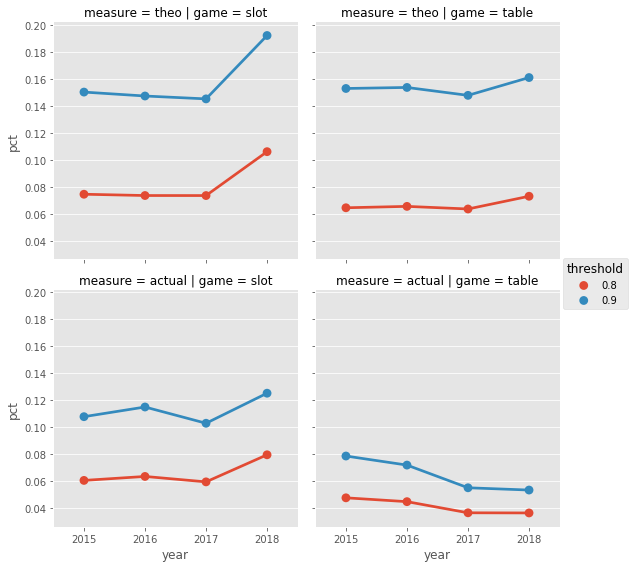In :
g = sns.factorplot(data=df_summary, x='year', y='pct', col='game', row='measure', hue='threshold',
ci=None, sharey=True, palette=sns.color_palette(["#d46a6a", "#801515"]))
g.set(ylim=(0))
g.set_titles("{col_name} {row_name}")
g.set_xticklabels(size=16, family='serif')
g.set_yticklabels(size=14, family='serif')
g.axes.yaxis.set_major_formatter(tkr.FuncFormatter(lambda x, p: "{:.1%}".format(x)))
g._legend.get_texts().set_text('80% of total')
g._legend.get_texts().set_text('90% of total')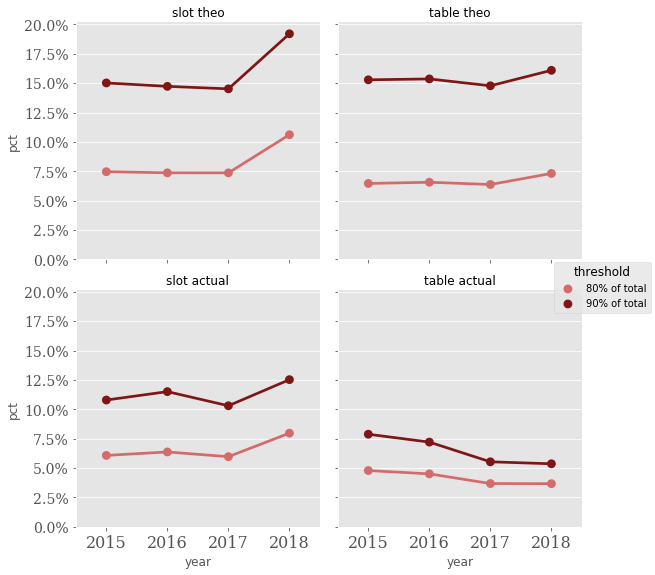#### This graph represents the same data we printed a few blocks up, because it's usually easier to see data in a graphical format¶

So let's interpret:

• the left column of plots are slots, the right column is table games
• the top row is theo, the bottom row is actual win/loss
• the lighter line is the 80% threshold, darker line is 90%
• on each plot, x-axis is the year and y-axis is the % of players responsible for either 80% or 90% of the business
• example: in 2015, it only took 7.5% of players to contribute 80% of total slot theo.
• that number was mostly steady the next 2 years, then spiked up to 10.6% so far in 2018.
• for table games, 90% of total actual win came from just just 5.4% of players!

Going back to the original question, yes, the casino relies on a small group of VIP customers to generate a huge percentage of its business. Instead of 80/20, it's more like 80/10 all the way down to 80/4, depending on slot vs table and theo vs actual.

OK, now we know what % of players contribute 80 or 90% of the business. Let's change the perspective a bit, and see the contribution from the top 10% of players. There is a lot of overlap with the previous function, but we need to do a little extra work to cut each subsection into deciles, then create a new dataframe for the chart data.

In :
# loop again to create plots --
# a little complicated because we need to perform the binning on each subset of data
# so we are repeating much of what the get_top_pct function does
for _measure in ['theo_sum' , 'actual_sum']:
df_chart = pd.DataFrame()
for _game in ['slot', 'table']:
for _year in [2015, 2016, 2017, 2018]:
df_base = df_slot_year if _game == 'slot' else df_table_year
sorted_series = df_base.xs(str(_year), level='year')[_measure].sort_values(ascending=False).reset_index(drop=True)
series_total = sorted_series.sum()
df_sorted = sorted_series.to_frame()
df_sorted['pct'] = sorted_series / series_total
df_sorted['cumulative'] = df_sorted.pct.cumsum()
df_sorted['decile'] = pd.cut(df_sorted.index, bins=10, labels=False)
df_sorted['year'] = _year
df_sorted['measure'] = _measure
df_sorted['game'] = _game
df_grouped = df_sorted.groupby(['year', 'measure', 'game', 'decile']).agg({'pct' : 'sum'})
df_chart = df_chart.append(df_grouped)
g2 = sns.factorplot(data=df_chart.reset_index(), x='decile', y='pct', col='year', row='game', kind='bar')
g2.fig.suptitle('Measure = {0}'.format(_measure.split('_').upper()))
g2.set_titles("{col_name} {row_name} " + _measure.split('_'))
g2.set_xticklabels(size=16)
g2.set_yticklabels(size=14, family='serif')
g2.facet_axis(0,0).yaxis.set_major_formatter(tkr.FuncFormatter(lambda x, p: "{:.0%}".format(x)))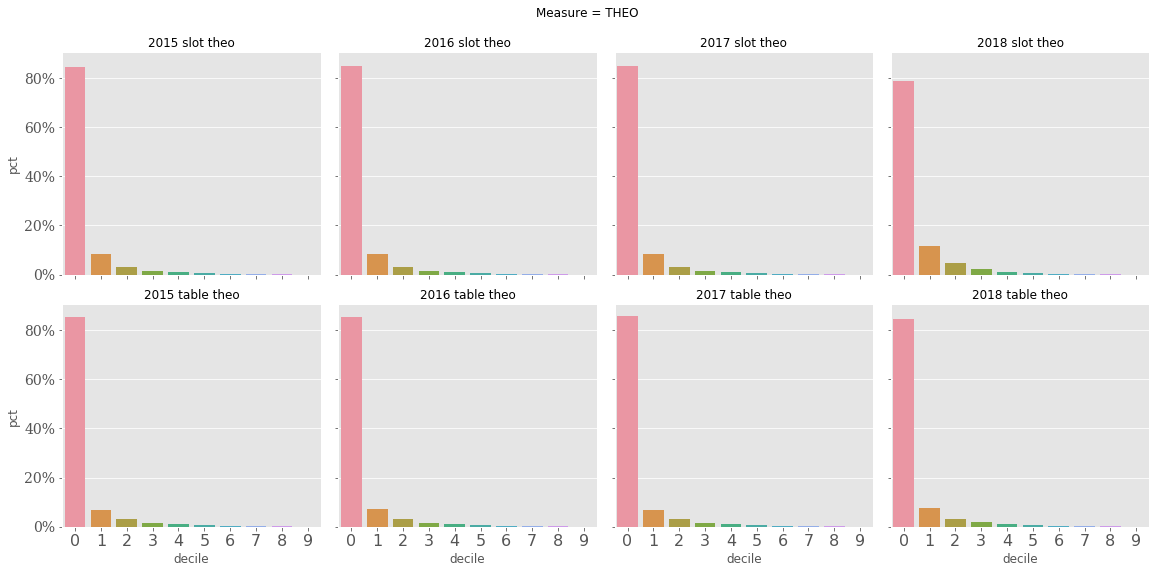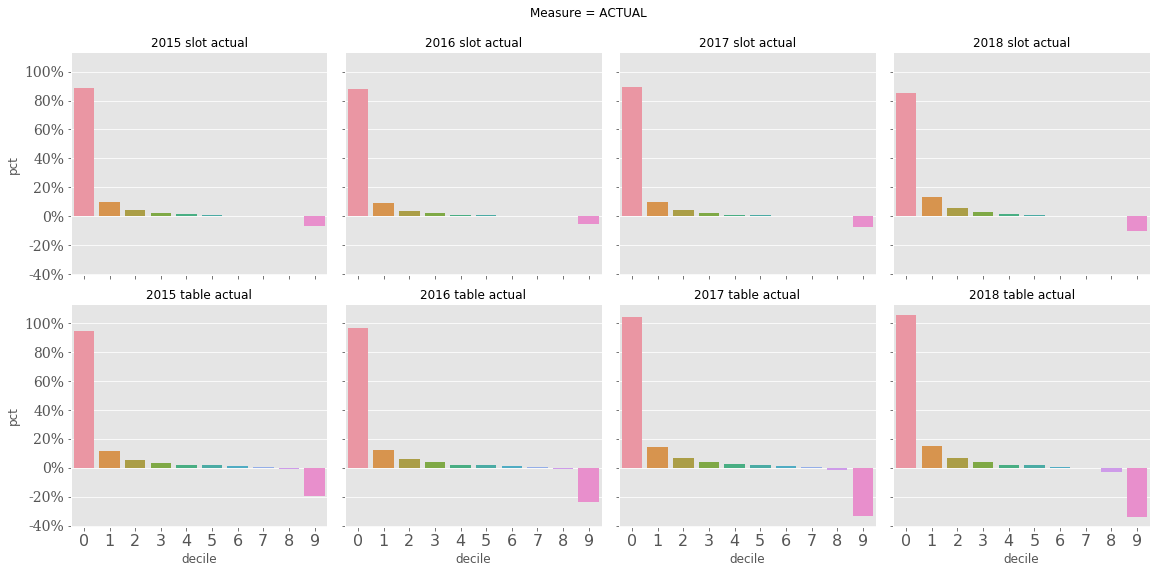This graph is sort of the inverse of the first graph. The first graph answered the question ____% of players contribute 80/90% of theo/win. This graph says the top 10% of players contribute ____% of win/theo. Because we divided each group into deciles, the left-most bar on each plot represents the top 10% of players.

## one final graph, let's profile the largest players¶

OK, one more graph -- let's just plot our top slot players each year by # of visits and theo per visit

In :
# push the index columns back to just regular columns
dfx = df_slot_year.reset_index()
# calculate avg_theo
dfx['avg_theo'] = dfx['theo_sum'] / dfx['days']
# a little trick to get the top X percent within each year, sorted by theo_sum
dfx_top = dfx.groupby('year', group_keys=False).apply(lambda x: x.nlargest(int(len(x) * 0.01), 'theo_sum')).drop(columns=['HashedPlayerKey'])
print(dfx_top.shape)
# show the first few rows

(8077, 5)

Out:
year theo_sum actual_sum days avg_theo
28450 2015 289165.677 229655.46 91 3177.644802
76829 2015 217366.913 362110.12 243 894.514045
733087 2015 212780.132 -6803.92 69 3083.770029
700971 2015 200489.864 227136.06 183 1095.573027
643409 2015 183721.744 142134.27 188 977.243319
586838 2015 176386.551 86631.56 138 1278.163413
464057 2015 159746.995 187120.52 264 605.102254
541750 2015 153783.499 266013.17 233 660.015017
767145 2015 152689.945 168002.88 51 2993.920490
668191 2015 147285.364 62774.10 111 1326.895171
393752 2015 144307.103 199656.37 137 1053.336518
763380 2015 136421.830 169140.41 93 1466.901398
348478 2015 135288.695 272667.97 160 845.554344
319037 2015 128648.243 41227.17 44 2923.823705
542199 2015 126414.008 -40198.30 134 943.388119

build the graph -- slightly different syntax than previous graphs

In :
# first, we do some calculations that will be used for additional dimensions in the graph
pointsize = abs(dfx_top['actual_sum'] / 2500) # must use abs() because we can't have negative-sized points
pointcolor = np.where(dfx_top['actual_sum'] > 0, '#E24A33', '#0F8935') # we will use an alternate color for winning players (negative actual_win)

# build the graph, the first line sets it up and second line maps various series to data elements
g3 = sns.FacetGrid(data=dfx_top, col='year', sharey=True, col_wrap=2, size=6)
g3 = g3.map(plt.scatter, 'days', 'avg_theo', s=pointsize, color=pointcolor)

# use a log scale for the y-axis
g3.axes.set_yscale('log')
g3.axes.set_yticks([100, 200, 500, 1000, 2000, 4000, 8000])
g3.axes.get_yaxis().set_major_formatter(tkr.ScalarFormatter())

# add a legend, in this situation we must create 'patches' to show in legend
loc_patch = mpl.patches.Patch(color='none', label='dot location: theo')
size_patch = mpl.patches.Patch(color='none', label='dot size: actual')
red_patch = mpl.patches.Patch(color='#E24A33', label='actual_win > 0')
green_patch = mpl.patches.Patch(color='#0F8935', label='actual_win < 0')
g3.axes.legend(loc='lower right', handles=[loc_patch, size_patch, red_patch, green_patch])

Out:
<matplotlib.legend.Legend at 0x10722ef0>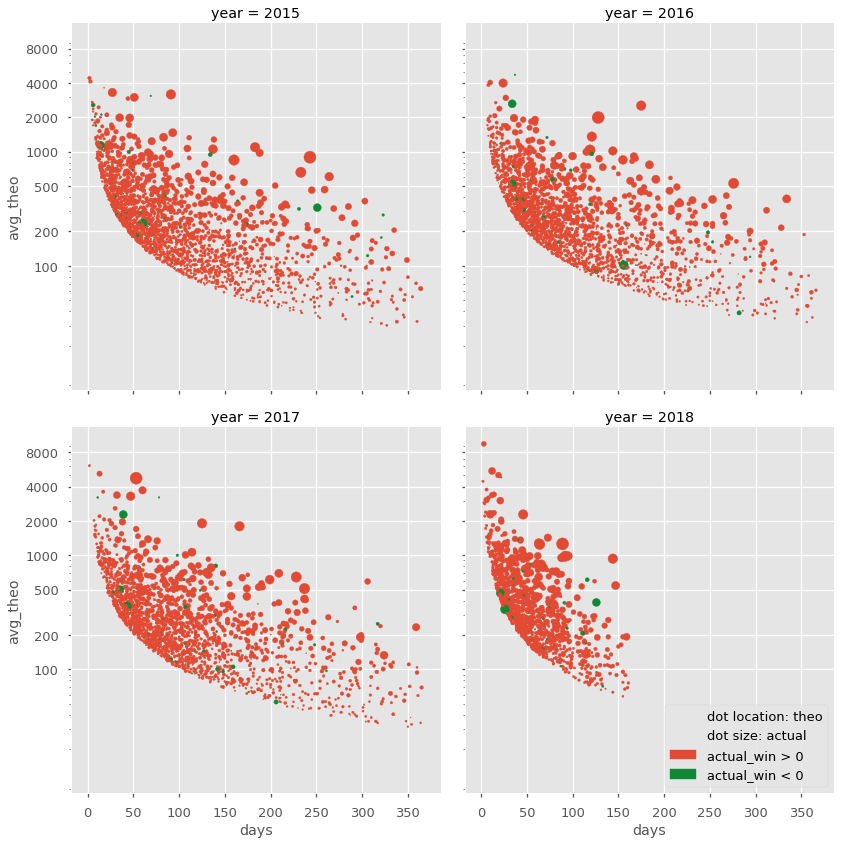Explanation of graph above: every dot represents one player among the casino's top 1% generators of slot theo (8077 dots total over the 4 years).

• The 4 plots represent the 4 years 2015-18 (analysis was done in June 2018).
• On each plot, every player's dot is placed according to # of visits during the year (x-axis) and average daily theo (y-axis).
• Note that the y-axis is logarithmic, so small vertical changes are actually quite large in dollars.
• The size of the dot is that player's total actual win/loss for the year.
• Because we can't have negative-sized dots, players who were net winners for the year are represented by green dots
• The curve naturally formed by the dots represent the minimum play needed to be among the top 1%: either very frequent visitation or very high average theo per day.